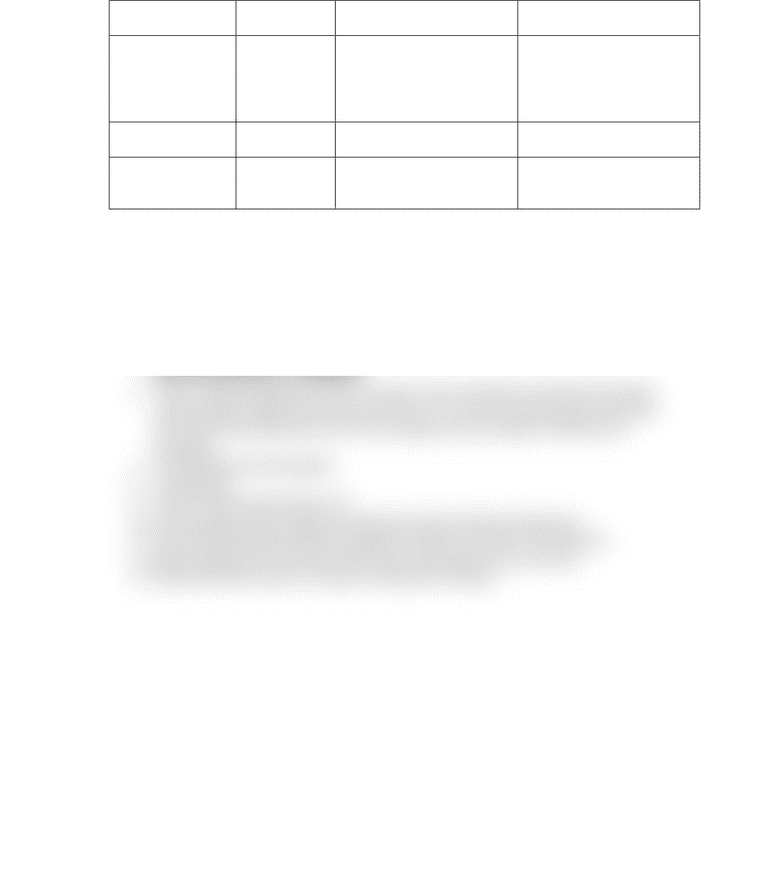# CHM 233 Study Guide - Final Guide: Chemical Formula, Wavenumber, Human Development Index

60 views1 pages
School
ASU
Department
Chemistry
Course
CHM 233
ProfessorIR signals
Determined
by
How it affects?
Also affected by
Frequency
(wavenumber)
Bond Strength
Mass
difference
Stronger bond (shorter
bond) gives higher frequency
Greater mass difference
gives lower frequency
Induction (higher frequency)
Resonance (lower frequency)
Shape
H-bond
More H-bond makes signal
Concentration (dilutes H-
bond)
Intensity
(% transmittance)
Bond dipole
Larger the dipoles gives
higher intensity signals (low
%transmitance)
Concentration (signals with
small bond dipole can add up
to make a significant signal)
How to obtain a structure from IR and MS spectra?
1. Obtain MW from (M)˙+ in MS spectrum.
2. Look for Cl or Br in MS spectrum (look at (M+2) signal and ratio (M):(M+2)
3. Look for N in MS (even mass vs odd mass) and IR (N-H bond)
4. Look for O in IR (O-H, C=O)
5. If the %relative abundance of 13C (M+1) is given, then calculate the number of Carbons
based on (M) and (M+1) calculations
6. If the % relative abundance of 13C is not given, then calculate the molecular formula by
subtracting the weight of heteroatoms (Cl, Br, N, O, etc) to the parent peak, then divide
the result of the subtraction over 12, this will give you the number of Carbons and
Hydrogens
7. Put molecular formula together
8. Calculate HDI
9. Look for all functional groups in IR
10. Draw possible isomers, based on molecular formula and functional groups
11. Look at the base peak and/other fragments to figure out losses in the molecule
12. Match fragments from the base peak and/or other peaks to the structures
13. Determine which isomer or isomers correspond to the data.
Unlock document

This preview shows half of the first page of the document.
Unlock all 1 pages and 3 million more documents.